# How many 17

How many fifths are there in four wholes? How many 1/5 are there in 4?

n =  20

### Step-by-step explanation: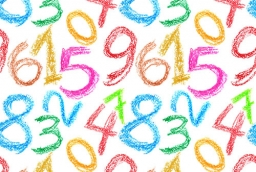Did you find an error or inaccuracy? Feel free to write us. Thank you!Tips to related online calculators
Need help to calculate sum, simplify or multiply fractions? Try our fraction calculator.
Do you want to perform natural numbers division - find the quotient and remainder?

## Related math problems and questions:

• 5 2/55 2/5 hours a week  mathematics,  3 3/4 hours a week   Natural sciences, 4 3/8 hours a week  Technology . how many hours does  he spend on social sciences if he spend 17 1/2 hours a week for the four subject?
• There 17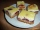There is 3/4 of a cake on a plate in Maria's kitchen.  Silvia sees the cake and eats 1/5 of the cake.  Then Franca takes 1/3 of what was there and shares half of her portion with Antonella.  What fraction of the cake is left?
• How many 14How many 1/2 cup serving are in a package of cheese that contains 5 1/4 cups altogether?
• Missing digitIf 4x/6x + 5/17 = 1, what digit does the x represents
• Camp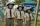In the camp are children. 1/2 went on a trip, 1/4 went to bathe, and 38 children remained in the room. How many children are in the camp?
• How manyHow many integers are greater than 547/3 and less than 931/4?
• StudentsThere are 2/6 girls out of 1050 students at the school. Of these, 4/5 have another sibling. How many girls are in school? How many boys are in school? How many girls do not have a sibling?
• Divide 11Divide the product of 4 and 5/8 by 1 1/2. Write your answer as a mixed number.
• Fractions and mixed numerals(a) Convert the following mixed numbers to improper fractions. i. 3 5/8 ii. 7 7/6 (b) Convert the following improper fraction to a mixed number. i. 13/4 ii. 78/5 (c) Simplify these fractions to their lowest terms. i. 36/42 ii. 27/45 2. evaluate the follow
• Evaluate expressionEvaluate expression using BODMAS rule: 1 1/4+1 1/5÷3/5-5/8
• Pupils 7There are 40 pupils in a certain class. 3/5 of the class are boys. How many are girls?
• Dividing walnuts into crates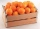There are 8 and 2 over 3 pounds of walnuts in a container, which will be divided equally into containers that hold 1 and 1 over 5 pounds. This would fill n and 4 over 18 containers. What is n?
• To improper fractionChange mixed number to improper fraction a) 1 2/15 b) -2 15/17
• RegroupingSubtract mixed number with regrouping: 11 17/20- 6 19/20
• Row for lunchThere are less than 66 people in a row for lunch. In front of Eva, there are 28 students. Behind Martin, there are 30 people. Between Eva and Martin, there are 17 people. How many students stand behind Eva if Martin stands in front of Eva?
• Sally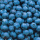Sally is making 15 individual blueberry cheesecakes. How many pounds of blueberries will be in each cheesecake if 5 1/4 pounds of blueberries are divided equally among them?
• Evaluate 17Evaluate 2x+6y when x=- 4/5 and y=1/3. Write your answer as a fraction or mixed number in simplest form.